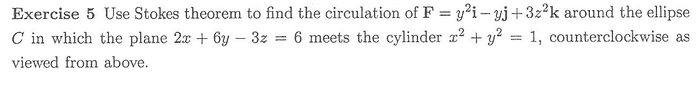# Stokes' Theorem Problem

## Homework Statement## Homework Equations

The double integral at S of the (curl of)F.n.

## The Attempt at a Solution

We find the the normal vector to the surface S is (2/7, 6/7, -3/7), right or not? We compute the curl of F which is -2y, right? Then, we calculate the dot product and we get 6y/7. Am I doing it right? If so, can someone tell me what to do next, and if not, can someone tell me how to properly solve it. I'm fairly new to the concept so please be patient with me.

## Answers and Replies

Then you'll perform the SURFACE integral of the dot product 6y/7 over the intersection of the plane and the cylinder, which is an elliptical disk. The integral can be converted to a DOUBLE integral with x and y in the circular disk x^2+y^2=1. The trick is to convert the area element dS of the elliptical to the area element dxdy in the circular disk. Intuitively dS*cos(theta)=dxdy, where theta is the angle of the intersection plane to the x-y plane.